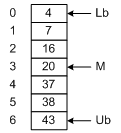# Arrays

Figure 1-1 shows an array, seven elements long, containing numeric values. To search the array sequentially, we may use the algorithm in Figure 1-2. The maximum number of comparisons is 7, and occurs when the key we are searching for is in A.Figure 1-1: An Array

 ```int function SequentialSearch (Array A, int Lb, int Ub, int Key); begin for i = Lb to Ub do if A(i) = Key then return i; return -1; end;```

If the data is sorted, a binary search may be done (Figure 1-3). Variables Lb and Ub keep track of the lower bound and upper bound of the array, respectively. We begin by examining the middle element of the array. If the key we are searching for is less than the middle element, then it must reside in the top half of the array. Thus, we set Ub to (M- 1). This restricts our next iteration through the loop to the top half of the array. In this way, each iteration halves the size of the array to be searched. For example, the first iteration will leave 3 items to test. After the second iteration, there will be 1 item left to test. Therefore it takes only three iterations to find any number.

This is a powerful method. Given an array of 1023 elements, we can narrow the search to 511 items in one comparison. Another comparison, and we're looking at only 255 elements. In fact, we can search the entire array in only 10 comparisons.

In addition to searching, we may wish to insert or delete entries. Unfortunately, an array is not a good arrangement for these operations. For example, to insert the number 18 in Figure 1-1, we would need to shift A...A down by one slot. Then we could copy number 18 into A. A similar problem arises when deleting numbers. To improve the efficiency of insert and delete operations, linked lists may be used.

 ```int function BinarySearch (Array A, int Lb, int Ub, int Key); begin do forever M = (Lb + Ub)/2; if (Key < A[M]) then Ub = M - 1; else if (Key > A[M]) then Lb = M + 1; else return M; if (Lb > Ub) then return -1; end; ```